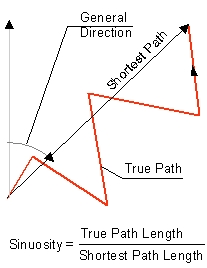Go to ET GeoWizards 12.x User Guide

Polyline Characteristics

Go to ToolBox Implementation

Calculates some characteristics of the polylines from a polyline dataset

Inputs:

• A Polyline feature class

Outputs:

• The results can be added to the input feature class or a new polygon feature class. All attributes of the original features are preserved
• New fields added to the attribute table
• ET_Sinous - the sinuosity of the polyline calculated as ratio  of the length of the polyline and the length of the line connecting the start and end points of the polyline. The value ranges from 1 (case of straight line) to infinity (case of a closed polyline). In case of infinity a 0 is recorded in the attribute table. See illustration below.
• ET_Vert - the number of vertices of the polyline
• ET_Dir - the general direction of the polyline - the direction in decimal degrees measured in North Azimuthof the line connecting the start and end points of the polyline (see illustration below).
• ET_Parts - the number of parts that the polyline has
• ET_HasArcs - if the polyline has true arc segments - 1 otherwise - 0
• ET_Closed - - if the polyline is closed - 1 otherwise - 0
• ET_Fract - the fractal dimension (indication of the complexity) of the polyline.  The value is between 1 and 2. The more complex the polylineis the larger  the fractal dimension will be.

Notes:

• Fractal Dimension of the polylines is calculated using the Box Counting method (1)

• Calculating the Fractal Dimension  is time consuming. If you don't need this characteristic, uncheck the option for faster processing.

Illustration:References:

1. Bourke, P., 1993. Fractal Dimension Calculator User Manual, Online. Available: http://paulbourke.net/fractals/fracdim/

Command line syntax

ET_GPPolylineCharacteristics <input_dataset>  {fractal_dimension}   {precision}

Parameters

Expression Explanation
<input_dataset> A Point feature class or feature layer.
{fractal_dimension} A Boolean indicating whether to calculate fractal dimension or not.
{precision} An Integer between 0 and 8 representing the number of places after the decimal point to be used.

Scripting syntax

ET_GPPolylineCharacteristics (input_dataset, fractal_dimension, precision)

See the explanations above:
<> - required parameter
{} - optional parameter